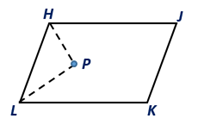Chapter 4.1, Problem 35EElementary Geometry For College St...

7th Edition
Alexander + 2 others
ISBN: 9781337614085

Solutions

Chapter
SectionElementary Geometry For College St...

7th Edition
Alexander + 2 others
ISBN: 9781337614085
Textbook Problem

The bisectors of two consecutive angles of ▱ HJKL are shown. What can you conclude regarding ∠P?To determine

To conclude:

The angle ∠P in the given parallelogram HJKL.

Explanation

Given:

The bisectors of two consecutive angles of HJKL are shown below.

Corollary:

A parallelogram is a quadrilateral in which both pairs of opposite sides are parallel.

In the given parallelogram IJKL.

 PROOF Statements Reasons 1. Parallelogram IJKL 1. Given 2. HJ-∥LK- 2. By the definition of a parallelogram 3. ∠LHP=∠H2 and ∠HLP=∠L2 3. Since HP and LP are angle bisectors of ∠H and ∠L 4

Still sussing out bartleby?

Check out a sample textbook solution.

See a sample solution

The Solution to Your Study Problems

Bartleby provides explanations to thousands of textbook problems written by our experts, many with advanced degrees!

Get Started

Prove each identity. sin(90)=cos

Trigonometry (MindTap Course List)

Which is the best graph of r = 1 − sin θ for 0 ≤ θ ≤ π?

Study Guide for Stewart's Multivariable Calculus, 8th

Find an expression for the mean of the probability density function .

Study Guide for Stewart's Single Variable Calculus: Early Transcendentals, 8th